# TRANSFORM SHAPES AND NODES

## MOVE :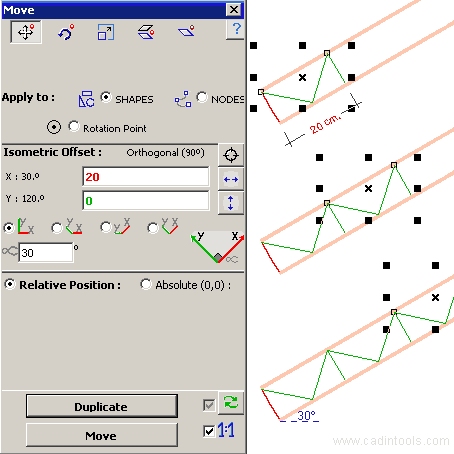•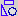Apply to Shapes : Move all selected shapes to X,Y.
•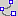Apply to Nodes : Move all selected nodes to X,Y (Relative Movement). <Example of Move Nodes>
•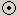Apply to Rotation Point : Move the Rotation Point of all selected shapes to X,Y.
• Isometric direction of X,Y: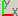Orthogonal,Left,Right and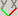Center. (Default = Orthogonal, angle = 0º)
• X, Y :
• Relative Position (Offset): is the distance to move.
• Absolute Position (from 0,0): is the Absolute Position desired for the active selection.

## ROTATE :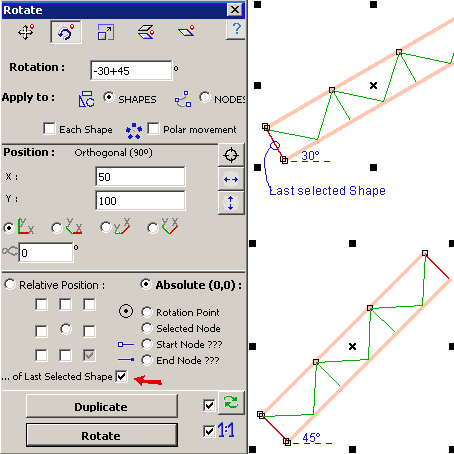• Rotation: Angle or rotation (degrees).
•Apply to Shapes : Rotate all selected shapes around X,Y.
•Apply to Nodes : Rotate all selected nodes around X,Y.
• Apply to Each Shape (Only for Relative Position):
•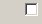(Default): Rotate the whole selection.
•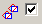Each Shape is rotated around its own Reference Position.
• Polar movement:
•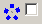(Default): Default rotation mode.
•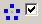Move selected shapes around the center of rotation (Polar Movement).<Example of Polar Movement>.
• Isometric direction of X,Y:Orthogonal,Left,Right andCenter. (Default = Orthogonal, angle = 0º)
• X, Y :
• Relative Position (Offset): is the offset to the "center of rotation", measured from the Reference Point of the active selection. After you Press [Rotate], Relative Position is toggled to Absolute Position.
• Absolute Position (from 0,0): is the Absolute Position of the "center of rotation" of the active selection.

## STRETCH :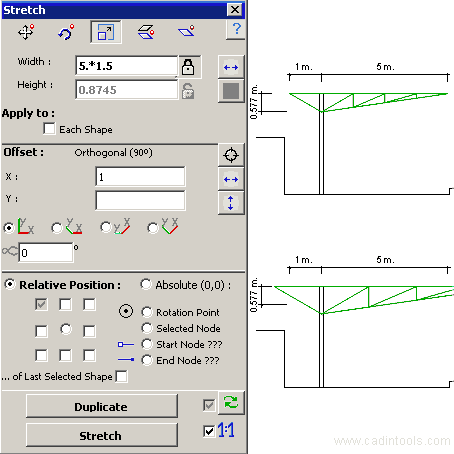• Width/Height : Size (Negative values are also accepted)
• Apply to Each Shape (Only for Relative Position):
•(Default): Stretch the whole selection.
•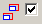Each Shape is stretched from its own Reference Position. <Example of Stretch Each Shape>
• Isometric direction of X,Y:Orthogonal,Left,Right andCenter. (Default = Orthogonal, angle = 0º)
• X, Y :
• Relative Position (Offset): is the offset to the "center of stretch", measured from the Reference Point of the active selection.
• Absolute Position (from 0,0): is the Absolute Position of the "center of stretch" of the active selection.

## MIRROR (FLIP)•,: Horizontal and Vertical mirror.
• Isometric : Isometric mirror (as seen in the above image), it is available only for the Orthogonal isometric direction.
• Apply to Each Shape (Only for Relative Position):
•(Default): Mirror the whole selection.
•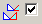Each Shape is mirrored from its own Reference Position.
• Mirror Text : (Default=Checked) :  If not Checked, text don't mirror. <Example of Mirror Shapes excluding Texts>
• Isometric direction of X,Y:Orthogonal,Left,Right andCenter. (Default = Orthogonal, angle = 0º)
• X, Y :
• Relative Position (Offset): is the offset to the "center of mirror", measured from the Reference Point of the active selection.
• Absolute Position (from 0,0): is the Absolute Position of the "center of Mirror" of the active selection.

## SKEW (TILT)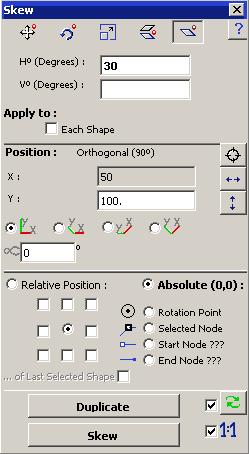• Hº(Degrees) / Vº(Degrees) : angle to skew.
• Apply to Each Shape (Only for Relative Position):
•(Default): Skew the whole selection.
•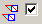Each Shape is skewed from its own Reference Position.
• Isometric direction of X,Y:Orthogonal,Left,Right andCenter. (Default = Orthogonal, angle = 0º)
• X, Y :
• Relative Position (Offset): is the offset to the "center of Skew", measured from the Reference Point of the active selection.
• Absolute Position (from 0,0): is the Absolute Position of the "center of Skew" of the active selection.

•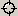Pick Point : Get the value for X and Y.
•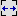Width: X = Width of the active selection. (If "each shape" is selected, the width belongs to the last selected shape)
•Height: Y = Height of the active selection. (If "each shape" is selected, the height belongs to the last selected shape)
•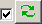Auto Refresh (Checked by default) : Refresh automatically the Absolute position of the active selection. Uncheck to lock an absolute position.
•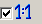Scale : (Checked by default) : Transform using the actual scale.